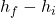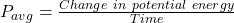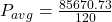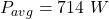## In 2 minutes, a ski lift raises four skiers at a constant speed to a height of 123 m. The average mass of each skier is 71 kg. What is the a

Question

In 2 minutes, a ski lift raises four skiers at a constant speed to a height of 123 m. The average mass of each skier is 71 kg. What is the average power provided by the tension in the cable pulling the lift

in progress 0
3 months 2021-07-28T15:10:11+00:00 1 Answers 49 views 0

The average power  provided by the tension in the cable pulling the lift is = 714 W

Explanation:

Given data

Mass = 71 kg

Change in height = 123 m

When the lift moves in upward direction then in that case kinetic energy is constant & only potential energy changes.

Change in potential energy Δ PE = m g ()

Δ PE = 71 × 9.81 × 123

Δ PE = 85670.73 J

Time = 2 min = 120 sec

So average power is given byTherefore the average power  provided by the tension in the cable pulling the lift is = 714 W